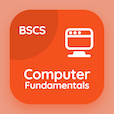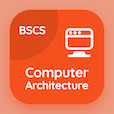Online CS Degree Courses

Database Systems MCQs

Database Systems MCQ - Topics

Practice Tuple Relational Calculus Multiple Choice Questions (MCQ Quiz), Tuple Relational Calculus quiz answers PDF to study database systems course for database systems online classes. Formal Relational Query Languages Multiple Choice Questions and Answers (MCQs), Tuple Relational Calculus quiz questions for top computer science schools. Tuple Relational Calculus Book PDF: relational algebra, domain relational calculus test prep for associates in computer science.

"The tuple relational calculus is a query language of type" MCQ PDF: tuple relational calculus App APK with procedural, nonprocedural, structural, and nonstructural choices for top computer science schools. Learn tuple relational calculus quiz questions for merit scholarship test and certificate programs for online degrees.

## MCQ on Tuple Relational Calculus Quiz

MCQ: The tuple relational calculus is a query language of type

Procedural
Nonprocedural
Structural
Nonstructural

MCQ: A tuple-relational-calculus expression may generate a/an

Finite relation
Infinite relation
Invalid relation
Composite relation

MCQ: A query expression in the tuple relational calculus is done as

{p| P(t)}
{p| t(p)}
{t| P(t)}
{p| t(t)}

### More Quizzes on Database Systems Book